## Friday, January 15, 2016

### I hope you find this blog enjoyable and helpful. Support it with a small donation.

In my last post I described a strategy for solving something I call the Triangle Game.  I supplied code and gave a quick description of its operation.  In this post I'll go into some of the finer details and explain the process of merging regions described by a list of vertices.  I've taken a subset of the game described in the last post and will use it to illustrate some points.

First a description of how the game works.  It's simple, add up all the numbers in all the triangles in the puzzle.  In the game below the numbers to add are in parentheses.  These follow a number that identifies the region.  For example 6(8) refers to region 6 that has a value of 8.  It's easy to see that regions 6, 8 and 9 form triangles, but there are other triangles as well.  The combination of region 6 and 7 also forms a triangle.  The key to solving this problem is to identify all the triangles by finding all possible combinations of regions and identifying which of these have shapes that can be described by a list of only three vertices.

 Puzzle - Planar Graph
You may ask why I didn't try to find triangular shapes and then add the triangles in that shape.  That seems easy, if I asked you to add the numbers in the triangle formed by the vertices 1, 4, and 6, you'd be easily able to tell me the answer is 8 + 10 + 1 = 19, but to do that you've used your vision system to identify which regions lie within this triangle.  When you're supplied with a list of vertices, connections, and straight lines with no specific information about geometry this task becomes harder.  I'll let you ponder this, in the image below find a programmatic way to determine that the blue triangle is located with the triangle formed by the three black dots.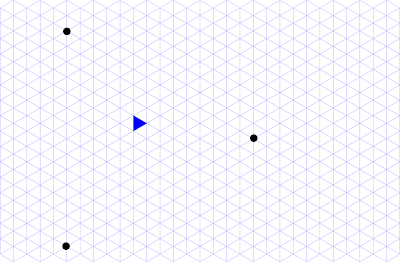How can you tell that the blue triangle is bounded by the 3 black dots?

I've done a little more reading since the first post and can describe things in more formal terms.  The puzzle is actually a planar graph, that is made up of the numbered regions and an external region that's ignored.  The funny thing is that the dual of the graph is more interesting.  The dual of a graph is made by placing a connection between regions every time an edge is encountered.  This usually includes a connection to a vertex in the external region, these are show below in grey, but in our case these can be ignored leaving only the graph shown in red.

Exploring this graph will identify all regions.  For example there are regions for each node 6, 7, 8, and 9.   There are regions for for each single edge (6, 7), (7, 8), and (8, 9), there are regions for each double connection (6, 7, 8), and (7, 8, 9), and finally there is a region for the single triple connections (6, 7, 8, 9).Dual of the puzzle graph

I'll run through the output of what happens when the above graph is entered into the software I wrote.

Firstly the regions of the graph that you supply are just echoed back.

Base regions ({id} =value= *vertices*)
count = 4

({6} =8= *1* *8* *7*)
({7} =10= *7* *8* *10* *6*)
({8} =1= *4* *6* *10*)
({9} =9= *5* *6* *4*)

The multi edge straight lines that you supply are echoed back.

Multi edge straight lines -*vertices*-
count = 2

-*8* *1* *10* *4*-
-*1* *5* *6* *7*-

Each region is inspected and each edge is added to a dictionary along with the region it is connected to. When completed you can easily see the regions that are connected by an edge.

edge list |*vertices*| -> {connected region id}
count = 10

|*4* *5*| -> {9}
|*8* *7*| -> {6}{7}
|*10* *6*| -> {7}{8}
|*1* *7*| -> {6}
|*4* *6*| -> {8}{9}
|*8* *1*| -> {6}
|*10* *4*| -> {8}
|*8* *10*| -> {7}
|*6* *7*| -> {7}
|*5* *6*| -> {9}

Compound regions are found by starting with base singular regions. Each edge of a region is checked against the list of edges above to see if any other region can be added to it. If so the new region is added to a set.  This prevents duplicates.  You will now have found all the compound regions containing 2 base regions.  This is repeated until all regions are found.

Compound regions ({id} =value= *vertices*)
count = 10

({8, 6, 7} =19= *7* *1* *8* *10* *4* *6*)
({7} =10= *7* *8* *10* *6*)
({8, 9} =10= *4* *5* *6* *10*)
({8, 7} =11= *7* *8* *10* *4* *6*)
({6} =8= *1* *8* *7*)
({8, 9, 6, 7} =28= *7* *1* *8* *10* *4* *5* *6*)
({8, 9, 7} =20= *10* *4* *5* *6* *7* *8*)
({6, 7} =18= *7* *1* *8* *10* *6*)
({8} =1= *4* *6* *10*)
({9} =9= *5* *6* *4*)

Each new region is checked to see if is triangular, if so, it can be added to the answer.  For example, region {6, 7, 8, 9} is described by the vertices (7, 1, 8, 10, 4, 5, 6), but looking at this circular list 3 vertices at a time we can reduce it to a triangle. 1, 8, and 10 are collinear, therefore 8 can be deleted. repeating this pattern we get the following (7, 1, 10, 4, 5, 6) - (7, 1, 4, 5, 6) - (7, 1, 4, 5) - (1, 4, 5)

Triangular Regions ({id} =value= *vertices*)
count = 6

({8, 6, 7} =19= *7* *1* *8* *10* *4* *6*)
({6} =8= *1* *8* *7*)
({6, 7} =18= *7* *1* *8* *10* *6*)
({8} =1= *4* *6* *10*)
({8, 9, 6, 7} =28= *7* *1* *8* *10* *4* *5* *6*)
({9} =9= *5* *6* *4*)

Sum of all the numbers in each triangular region = 83

The value of these triangles is added together to find the answer.  In this case it's 83.

So far I've mentioned merging or adding regions together.  What does that actually mean?  As all the regions have a numerical value or worth, the first step is to just add these together.  To put it simply imagine you and your neighbour wanted to join your blocks of land, and you wanted to know how many trees would be on the new block.  If you previously had 2 and they had 5, the new block would have 7 trees.  This is great but it doesn't describe the shape of the new property.  For that you need to supply a list of vertices that describe the boundary.

If we're smart about it and describe each boundary with a circular list in a consistent clockwise or anticlockwise manner we'll make things easier for later.  For the examples going forward I'll use the letters R (red), G (green), and B (blue) to describe the regions.  The two regions below can be described as

R = [a, b e, f]
G =  [b, c, d, e]Two connected regions

To add them together a shared edge needs to be found, in this case that's b-e.  The circular vertex lists describing each region are now rotated until this shared edge is at the end if it isn't already.

R = [f, a, b, e]
G = [c, d, e, b]

Joining the vertex lists is done by removing the last vertex from each list and then concatenating them.  As the lists have been defined with a consistent direction of rotation, the joining process is simple.

{R, G} = [f, a, b, c, d, e]Regions joined on edge b-e
What if the regions have more than one shared edge?  The same process can still be used.  If R = [a, b, g, h, e, f] and G = [b, c, d, e, h, g].Two regions that share more than one edge
Joining them on the edge h-e will give the new region {R, G} = [f, a, b, g, h, g, b, c, d, e].  This new region is a bit strange though.  It has a fold.  These can be identified by vertices that double back on themselves. In this case g, h, g.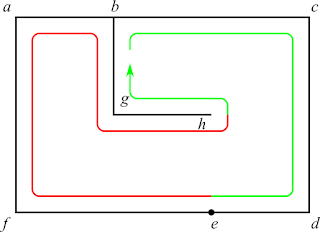Join regions on edge h-e
Removing this fold can be done by replacing the vertices g, h, g with just g to get {R, G} = [f, a, b, g, b, c, d, e]Remove folded edge g-h
Likewise the fold b, g, b, can be removed to give {R, G} = [f, a, b, c, d, e]Remove folded edge b-g
We've show that joining multiple regions is possible, but what if they're not consecutive edges.  In the structure below, if regions R and G are joined, that can be done on the the edge b-h or the edge k-e.  Depending on the edge chosen, the result will be different.  This happens because the outline of the internal region made when R and G are added still needs to be connected to the outer border.  Imagine tracing the border with a pencil and not lifting the pencil.  You have to pick a way to get between the outer and inner borders.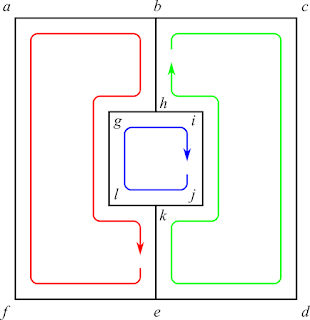3 Region Graph
By joining the regions on the edge k-e, there's an implicit decision to get between the inner and outer borders via the edge b-h.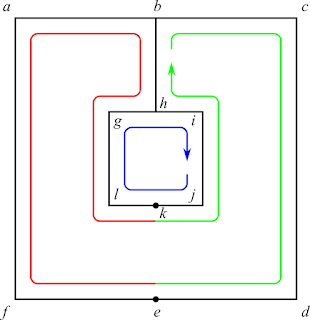Join regions R and G on edge k-e
Adding the region B to region {R, G} can now be done.  It will require removing multiple folds that form a tree.  A recursive process of removing folds will ultimately yield the simplest outer border.Add region B to region (R + G) on edge l-k
.Remove folded edge g-l
.Remove folded edge g-h
.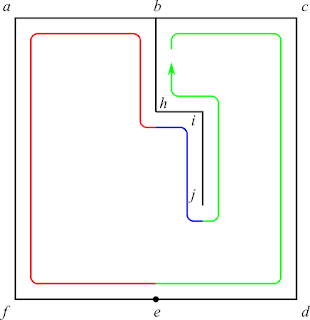Remove folded edge k-j
.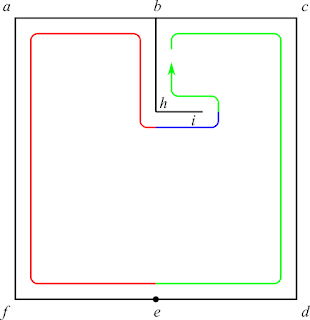Remove folded edge j-i
.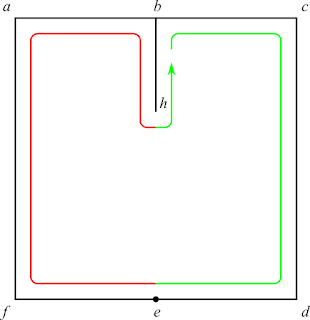Remove folded edge h-i
.Remove folded edge b-h

A few observations I made while exploring region merging are worth mentioning.  In the graph below, if the only regions defined are [a, d, f, c], [a, b, e, d], and [c, f, e, b] the graph can only be assembled in one way.  That will give an internal region of [f, d, e].4 region graph defined when 3 regions supplied

However, in the two graphs below if only the regions [a, b, h, g, l, k, e, f] and [b, c, d, e, k, j, i, h] are defined there are 2 configurations that will give different graphs.  One will contain an internal region of [g, h, i, j, k, l] and the other will contain an internal region of [f, e, d, c, b, a].  So to completely specify the graph all of the regions need to be specified in this case.  I don't know what differentiates the two scenarios.  Maybe the case of two regions with multiple non consecutive shared edges is the only example of this effect.  I don't know. I haven't put much thought into it.  I just thought that it was worth mentioning.3 region graph defined by 2 regions - configuration 1
.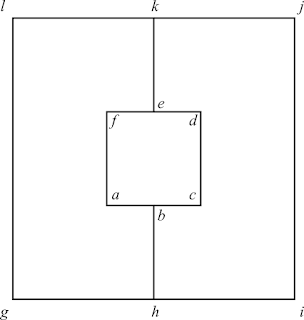3 region graph defined by two regions - configuration2

I'll leave you with a few resources you should probably look at before starting on any project like this.  There is a standard notation to describe planar graphs called a "combinatorial map".  There is also a standard, ISO 10303, that explains how to exchange boundary representation of models. Finally there is the Computation Geometry Algorithms Library (CGAL).  It's probably overkill for this problem, but it's still worth a look.